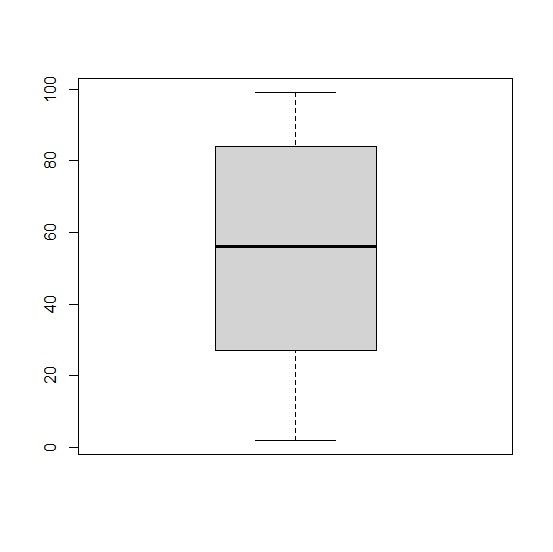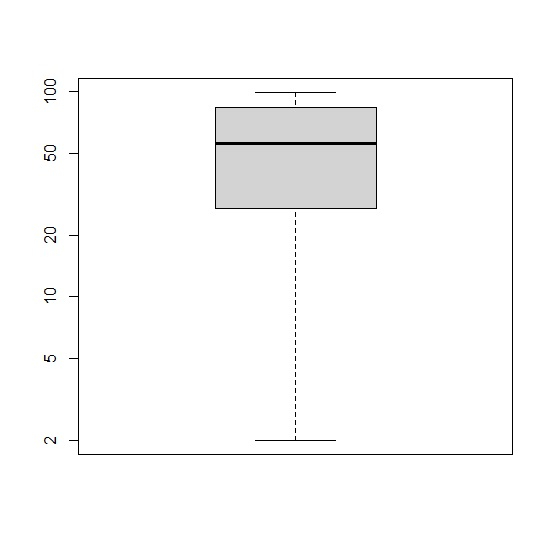# How to create a boxplot with log of the variable in base R?

To create a boxplot with log of the variable in base R, we need to use log argument within the boxplot function but we need to carefully pass the Y-axis inside the function because the values of the boxplot are plotted on the Y-axis. For example, if we have a vector called x then the boxplot of log of x will be created as boxplot(x,log="y").

## Example

x<-sample(1:100,50)
boxplot(x)

## Output## Example

boxplot(x,log="y")

## Output